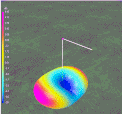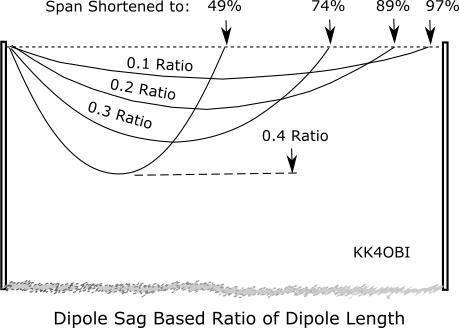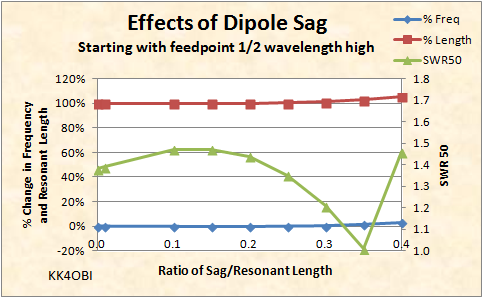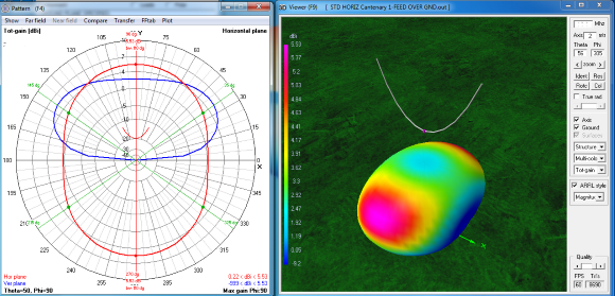This is a discussion about sag in a dipole and the resulting changes of antenna characteristics and far field radiation patterns.

 Navigation Menu Center-fed Bent-Dipoles Horizontal Lateral Vertical OCF EFHW Slow-Wave Other Topics Home One of the interesting things about  the natural sag of a wire, rope or chain between two supports is that it forms a unique shape, a catenary curve.The mathematics to describe this catenary curve defied solution until Calculus was invented. To this day, differential equations, integrals and specialized software are how engineers design bridges and arches. There is no direct solution. In the world of ham radio a straight dipole antenna is rare because wire sags and tubing droops. And of course, we can't mathematically model this because the demands for catenary calculations are beyond the capabilities of the Numerical Electromagnetics Code which is the core of our antenna modeling programs.  Antenna models look at practical solutions by using only straight lines, arcs or helices. The antenna sag studies in this section use a straight-line approximation of the curve. By employing specialized catenary calculation programs, a set of four equations were developed to describe the antenna sag equal to 10, 20, 30 and 40 percent of the length of a straight resonant wire.  The span of each curve was divided into ten segments and the sag-to-length values on each curve were normalized and averaged.  This set of values is used to create a point-to-point approximation of a catenary curve in antenna modeling software.Figure 1 The key to creating a dipole sag model is to use the software optimization function.  Fortunately, there is one equation that can directly calculate the width of the catenary span between two poles knowing the length of the wire and the height of the poles. To run the model you merely set a sag ratio.  The program will experimentally try a length of wire, find the catenary span for that sag ratio, divide the span into ten plotting points, form the point-to-point cantenary curve, test it for minimum SWR and +/-j reactance and repeat automatically until the best resonant length of wire is found. 4NEC2 Antenna Model: Here With this model it is possible to answer the question: What are the effects of dipole sag? In Figure 1, the first thing that jumps out is how gain goes down (Green Line) with the smallest amount of sag.  No matter how minute the sag you will never achieve maximum gain.Figure 2 A second oddity is the Z Ohms impedance (Blue Line) goes up until sag reaches around 25% of the wire length, then starts down.  Very unusual. On speculatimg about this remember that the feed point starts at 1/2 wavelength over average ground and goes toward ground with increasing sag. Another counter-intuitive characteristic is that the % Radiation Efficiency (Red line) rises with increasing sag.  An examination of the vertical and horizontal components of the far field radiation pattern suggests that as the sag becomes greater than 10%, the vertical radiation increases and adds to the total. The real surprise is that you can tune a dipole by the amount of sag!Figure 3 In Figure 3 follow the Green line for SWR50.  Sag de-tunes a dipole up to 23% sag. Then, at 35% sag, there is a perfect 50 Ohm match for coaxial cable.  Remember, the model is over a mathematical  "average ground" so the exact % sag will be controlled by height and earth conductivity. Sag seemingly violates one of the eternal truths of bent dipoles: Bending a dipole shortens the radiating length causing the frequency to rise.  Curiously we see that sagging a dipole has almost no effect on the radiating length and frequency.  Looking at the Blue line above it can be seen that frequency does not begin to rise until you reach the 35% resonant point where a slight lengthening (Red line) is necessary stay on frequency.  This phenomena offers a down-and-dirty way for field day tuning. Just droop the dipole for match and don't worry about tuning. Well, maybe worry about radiation pattern.Figure 4 Far Field Radiation Pattern at 35% Dipole Sag As seen in Figure 4, the usual long, lean, figure-8 shape of a dipole is gone.  Now it is shorter and considerably plump. SWR is 1:1 vs 1.38 for a standard dipole. Gain is 5.53 dBi  vs. 7.32 dBi of a standard dipole End Nulls of -2.7 dBi are gone.  Now there is +0.22 dBi Gain off the ends because the vertical character of the ends of the arms produces gain that completely fills in the usual nulls. Radiation Efficiency is slightly better at 75.2% vs 74.5%. The angle of greatest radiation (Purple on 3D picture) is higher, now at 40° up from horizontal vs 30° for standard dipole. The beam width (at 3Db down) is a generous 110°. This concludes the results of the antenna model for a cantenary curve which was used to answer the question about changes in dipole characteristics related to sag. Dick Reid, KK4OBI at QSL.net